# Performance of a Field of Geothermal Probes to Support the Air Conditioning Plant of a Public Building Powered by Water/Water Heat Pumps

Performance of a Field of Geothermal Probes to Support the Air Conditioning Plant of a Public Building Powered by Water/Water Heat Pumps

M. Cucumo  V. Ferraro* D. Kaliakatsos M. Mele G. Barci

University of Calabria - DIMEG - Via P. Bucci, Cubo 44C – 87036 Rende (CS) - Italy

Barci Engineering - Via G. Falcone 14, 87040 Montalto Uffugo (CS) – Italy

Corresponding Author Email:
vittorio.ferraro@unical.it
Page:
S535-S544
|
DOI:
https://doi.org/10.18280/ijht.34S248
|
Accepted:
|
Published:
31 October 2016
| Citation

OPEN ACCESS

Abstract:

The design of a field of geothermal probes coupled to a heat pump of the water/water type supporting the air conditioning plant of the Rectory of the University of Calabria, is presented. The convenience of a geothermal plant is linked to the prevailing weather conditions, i.e. it is of greater benefit when the location temperatures are harsh. Although the location of the installation is not characterized by a very low project external temperature (-3.2 °C), the heat exchange gradient generated in both Cooling seasons is enough at the ground to carry out the task of a hot/cold well. For the sizing of geothermal heat pump systems, reference is made to the UNI 11466: 2012 and ASHRAE recommendations. The water/water heat pumps are designed for specific values of the outlet water and in return reaching COP values next to 4.4. Their coupling with a properly sized SGV system keeps the value of the COP constant for the entire cooling season. By sizing performed for the object of the case study, the building was achieved with a total length of variable probes between 4200 m and 4800 m according to the theorized wheelbase. Taking into consideration the cost items and the long-term system performance, it can be said that the optimal solution is the use of 28 probes each 150 m in length. This result was later validated by the simulations conducted with the TRNSYS software from which was found a water return temperature to the HPs always below the 30 °C required, resulting in further improvement of performance of the machine. In the paper we present the calculation results according to the standards and their comparison with those obtained with the TRNSYS 17 software.

Keywords:

Performance, Geothermal probes, Building air conditioning, Water/water, Heat pump.

1. Introduction

The convenience of a geothermal system is typically linked to the external climate, which is more beneficial when the location climate is harsh. The design temperatures for the locations of the plant at Arcavacata of Rende (CS), Italy, are -3.2 °C for the winter season and 33 °C for the summer. The heat exchange gradient generated in both cooling/heating seasons is enough at the ground to carry out the hot/cold well task.

The work presents the field dimensioning of geothermal probes and the heating and cooling effect of the solid matrix of the soil, following its continuous use.

The parametric simulation results with the ASHRAE method  and with the software TRNSYS 17  are presented and, finally, the results about the suitability of employing different types of coupling for different lengths of the probes, are analyzed critically. Special attention is also paid to the phenomenon of temperature drift.

2. Ashrae Method

The UNI 11466: 2012  defines the design criteria and the calculation procedures for the determination of the design performance of the geothermal heat pump systems. The standard also allows determination of the average monthly temperature of the heat transfer fluid side land needed to determine the energy performance of the heat pumps for the energy certification of buildings.

The calculation process currently used refers to the methodology developed by Ingersoll et al.  and subsequently applied and implemented by Kavanaugh and Rafferty , indicated for convenience by the name of the “ASHRAE method”.

The rule applies to geothermal plants employing a heat pump with secondary fluid, used for winter and summer air conditioning and for the production of sanitary hot water by heat exchange with the ground and is based on heat transfer in steady state. It is a simplified method and allows valid values to be obtained for a first sizing of the sensors field. The total length of the probe field is calculated, according to the season taken into consideration, namely the winter or summer, with the following relations:

$L_{h}=\frac{Q_{a} R_{g a}+\left(Q_{l h}-W_{h}\right) \cdot\left(R_{b}+P L F_{m, h} R_{g m}+R_{g d} F_{s c}\right)}{T_{g}-\left(\frac{T_{w i}+T_{w o}}{2}\right)_{h}-T_{p}}$(1)

${{L}_{c}}=\frac{{{Q}_{a}}{{R}_{ga}}+\left( {{Q}_{lc}}-{{W}_{c}} \right)\cdot \left( {{R}_{b}}+PL{{F}_{m,c}}{{R}_{gm}}+{{R}_{gd}}{{F}_{sc}} \right)}{{{T}_{g}}-{{\left( \frac{{{T}_{wi}}+{{T}_{wo}}}{2} \right)}_{c}}-{{T}_{p}}}$ (2)

The subscripts “c” and “h” respectively identify the length of the probes necessary to meet the power for cooling and for heating. The total length of the probes to be installed, corresponds to the larger of the two resulting for the heating and the cooling. The other terms that appear in the equations, however, are identified as follows: Qa is average annual power exchanged with the ground (positive in the case of heating, negative in case of cooling); Qlc and Qlh  are the thermal design loads for cooling (negative) and the heating (positive); Wc and Wh represent the electric power absorbed by the compressor of the heat pump to meet the Qlc and Qlh loads; Rb is the thermal resistance per unit length of the probe; Rga, Rgm and Rgd are the equivalent thermal resistance per unit length of the ground for annual, monthly and daily boost, PLFm,c/h  the dimensionless factor of capacity in the project month, Fsc the loss dimensionless factor related to the possible short circuit in the heat sensor between supply and return pipe; Tg is the undisturbed ground temperature not influenced by the presence of the probe; Twi and Two are the inlet and outlet temperatures of the fluid that feeds the geothermal probes in the design conditions, Tp the penalization temperature which summarizes the thermal influence between the probes through the ground (positive warming, negative in the case of cooling).

The design parameters required for the calculation of these lengths are then the temperature of the undisturbed soil, the flow temperature and return related to the field of probes, soil properties, i.e. conductivity and thermal diffusivity, and heat-transfer pipe (available, number per well, diameter, thermal characteristics of the filling); characteristics of probes field (number, distance, circuitry, disposal), thermal design loads and equivalent hours at full load.

A division of the heat exchange regions in two distinct areas is adopted in eq. (1) and (2): one associated with the thermal resistance of the soil around the well and the other that of the well containing the filling material, the vertical and the fluid probes heat transfer. While in the first region the temporal pulses are taken as a reference, in the second the resistance considered is associated with the heat transfer fluid, to the pipes and to the pit filling material. It is referred to as a transient phenomenon in the first case and in the second stationary. The calculation of the soil resistance requires knowledge of the time period for which the heat pulse is applied for which three different types can be identified: annual net heat flows; monthly thermal flows inherent to the month of the project and maximum thermal fluxes achieved during the project day. This leads, therefore, to the calculation of Rga, Rgm and Rgd resistances by applying the cylindrical source method .

The average annual power exchanged with the ground is calculated using one of the following relationships:

${{Q}_{a}}=\frac{\sum{{{Q}_{lh}}\cdot \left( 1-\frac{1}{\overline{CO{{P}_{h}}}} \right)\cdot {{\tau }_{h}}}+\sum{{{Q}_{lc}}\cdot \left( 1+\frac{1}{\overline{CO{{P}_{c}}}} \right)\cdot {{\tau }_{c}}}}{8760}$ (3)

${{Q}_{a}}=\frac{{{C}_{fc}}\cdot {{Q}_{lc}}\cdot {{\tau }_{c}}+{{C}_{fh}}\cdot {{Q}_{lh}}\cdot {{\tau }_{h}}}{8760}$ (4)

where COPh and COPc are the average seasonal values of the coefficient of performance of the heat pump.

The two equations allow, with discrete approximation, the same result to be reached; the difference lies in the dependence of Eq. (4) by Cfc e Cfh vestments that are computed by linear interpolation from the values tabulated as a function respectively EER (Energy Efficiency Ratio) and COP (Coefficient Of Performance) of the heat pump (Table 1). The COPh values and COPc must be calculated using the detailed data sheet of the heat pump as a function of the flow and return temperatures (Twi and Two) calculated based on the energy demands of the building obtained using the UNI TS 11300-4 . The load factor/slicing of the project month, regardless of the season climate, is defined as:

$PL{{F}_{m}}=\frac{\sum\limits_{1}^{24}{Thermal\,Hourly\,Load\cdot hours}}{Peak\,\,Load\cdot 24}\cdot \frac{\text{Monthly days of operation}}{D\text{ays per month}}$ (5)

As can be seen, PLFm,c/h is very similar to a utilization factor and is therefore dimensionless. The equation (5), suitably simplified becomes: COPc

$PL{{F}_{m,c/h}}=\frac{Equiv.\,\,hours\,(at\,peak\,load)\,\,\text{in project month}}{\text{Hours in}\,\text{a day}\cdot \text{Days per month}}$ (6)

For simplicity of calculation, the need to know directly the values of Wc and Wh, is overcome using in Eq. (1) the difference between the loads of Qlc project and Qlh and the values of and, provided by the following simplified relationship:

$\left(Q_{l c}-W_{c}\right)=-Q_{l c} \cdot\left(1+\frac{1}{C O P_{C}}\right)$(7)

$\left(Q_{l h}-W_{h}\right)=Q_{h} \cdot\left(1-\frac{1}{C O P_{h}}\right)$(8)

At the peak load, the values of the heat pump coefficient of performance are :

$C O P_{h}=\overline{C O P_{h}} \cdot C_{f h}=\overline{C O P_{h}} \cdot\left[0,57+0,16 \cdot \ln \left(C O P_{h}\right)\right]$(9)

$C O P_{c}=\overline{C O P_{c}} \cdot C_{f c}=\overline{C O P_{c}} \cdot\left[0,57+0,16 \cdot \ln \left(C O P_{c}\right)\right]$(10)

The undisturbed soil temperature can be calculated theoretically through the application of the law on the propagation of heat from the surface of the soil in depth.

$T_{g}=T_{0}-\Delta T_{0} \cdot e^{\left(-\frac{z}{\delta}\right)} \cdot \cos \left[\omega\left(t-t_{0}-\frac{z}{\delta}\right)\right]+T_{g e o}\left(z, \lambda_{g}\right)$(11)

where Tg is the temperature of the subsoil to the time “t” and the depth “z”; T0 is the average annual temperature of the locality, ΔT0 the variation of the amplitude of the sine wave of the surface temperature (min/max), t0 the number of the day which corresponds to the minimum ambient temperature (January 1 = 1), T the period of the sinusoidal pulse (365 days),$\omega=\frac{2 \pi}{T}$ the heat wave pulse, $\delta=\sqrt{\frac{\alpha_{g} \cdot T}{\pi}}$ the depth o f penetration of heat wave, $\alpha_{g}=\frac{\lambda}{\rho \cdot c}$ the thermal diffusivity of the soil, λg the thermal conductivity of the ground, ρg the density and cg the ground specific heat.

The resulting temperature from this equation corresponds to a development of the temperature of the soil as a function of depth and of the season of reference. It is easily seen that at a depth of more than 15 m the surface temperature variation does not influence the value found in depth. In Eq. (11) the effect of the geothermal temperature gradient (3 °C per 100 m) has been taken into account which alters the temperature of the subsurface due to the heat from the earth's core and of the variation of the thermal conductivity of the soil due to the different materials that characterize it. The latter generates zones of discontinuity which, in the contact zones between the different layers, leads to significant changes in temperature that develop over short variations in depth.

Once identified the initial temperature of the undisturbed ground, it will be affected by the heat exchange due to the presence of geothermal probes. The distance between them can affect the optimum operation of the probes because they can interfere with each other when too close. To offset this effect, the penalty temperature Tp is inserted in Eqs. (1) and (2), which summarizes the mutual interference of thermal fields of the probes through the ground, penalizing the thermal jump between the fluid and the undisturbed ground. The penalty temperature assumes low values if the summer and winter loads are similar in form, while becoming higher if the required power is very different during the summer or winter operating system. This term implies, therefore, an increase in the required depth, for the consequent reduction in the thermal head, and is determined by the system activation time, the arrangement and the distance between the probes.

There are two methods for the calculation of this parameter. It can for instance be used for systems with heat load in the peak period around 350 kW, a tabular method of obtaining an approximate value , or you can use eq. (12) where the probe is based on the accumulation of annual heat in the surrounding soil.

$T_{p}=T_{p 1} \cdot\left(\frac{1 \cdot N_{4}+0,5 \cdot N_{3}+0,25 \cdot N_{2}+0,1 \cdot N_{1}}{N_{t a t}}\right)$(12)

where N1, N2, N3 and N4 is the number of probes respectively surrounded by 1, 2, 3 or 4 probes; Ntot is the total number of probes; Tp1 is the of a single probe penalty temperature surrounded by other probes on all sides, be calculated as follows:

$T_{p 1}=\frac{Q_{c c c}}{\rho \cdot c_{p} \cdot d_{s}^{2} \cdot \ell}$(13)

where Qacc is the heat accumulated after 10 years of operation using the solution of the linear source and considering a cylinder with a diameter of 8-10 meters, ρ the density, cp the specific heat of the land, ds the distance and $\ell$ the length of the probes.

To calculate the equivalent resistance of the soil, using the solution proposed by Carslaw and Jaeger , which puts in relation the time during which the heat exchange occurs with the outside diameter of the probe and the diffusivity of the soil through a Fourier number:

$F o=\frac{4 \cdot \alpha_{g} \cdot \tau}{d_{b}^{2}}$(14)

where αg is the thermal diffusivity of the soil, db [m] outer diameter of the drilling and τ the period characterizing the impulse. For a system of this type, the hypothesis that undergoes three heat flux pulse is made, relating to the three periods of time:

• τ1 = 10 years (3,650 days);
• τ2 = 10 years+1 month (3,650 + 30 years);
• τf = 10 years+1 month +6 hours (3,650 + 30 + 0.25).

Rewriting Eq. (14) for the three different pulse periods, you obtain three different values of Fo:

$F o_{f}=\frac{4 \cdot \alpha_{g} \cdot \tau_{f}}{d_{b}^{2}}$

$F o_{1}=\frac{4 \cdot \alpha_{g} \cdot\left(\tau_{f}-\tau_{1}\right)}{d_{b}^{2}}$(15)

$F o_{2}=\frac{4 \cdot \alpha_{g} \cdot\left(\tau_{f}-\tau_{2}\right)}{d_{b}^{2}}$

These values of Fo are selected to the resistances which refer to the soil surrounding the hole by calculating the “G-factor” based on the theory of the cylindrical source. The three values of G-factor, Gf, G1 and G2 are obtained by Eq. (16), valid for Fo > 2 numbers; for values of Fo < 2 the value is obtained for via Graphic .

$G=0.0758 \cdot \ln (F o)+0.1009$(16)

The three resistors Rga, Rgm, Rgd therefore be defined as follows:

$R_{g m}=\frac{G_{1}-G_{2}}{\lambda_{g}}$(17)

$R_{g m}=\frac{G_{1}-G_{2}}{\lambda_{g}}$(18)

$R_{g d}=\frac{G_{2}}{\lambda_{g}}$(19)

The term, Rb, is the thermal resistance per unit length between the fluid and the ground, at the outer surface of the probe, in contact with the ground. This thermal resistance is calculated as:

$R_{b}=R_{p p}+R_{g r}+R_{T}$(20)

where Rpp is the overall thermal resistance of the pipes in which the fluid flows, Rgr is the thermal resistance of the filling jet and RT is thermal resistance of the steel jacket.

The configuration chosen for the probes is that dual U. In this case the thermal resistance probes-soil is calculated by the expression :

$R_{g r}=\frac{1}{2 \pi \cdot \lambda_{g r}}\left[\ln \left(\frac{d_{b}}{d_{p o}}\right)-\frac{3}{4}+\left(\frac{d}{d_{b}}\right)^{2}-\right.$$-\frac{1}{4} \ln \left(1-\left(\frac{d}{d_{b}}\right)^{8}\right)-\frac{1}{2} \ln \left(\frac{\sqrt{2} \cdot d}{d_{p o}}\right)-\frac{1}{4} \ln \left(\frac{2 \cdot d}{d_{p o}}\right) ]$(21)

where d is the distance between the double U-shaped pipes and λgr the conductivity of the hole fill material.

3. Case Study

The object of study building, seat of the Rectory of the University of Calabria (Italy), has a square base with each side about 21 m. It rises to 18 meters above ground on 5 floors, as well as having a semi-basement. The semi-basement floor is partially heated while the 5 floors are fully air-conditioned except for the stairwell. The heat output of the resulting project will be up to 177 kW for summer air conditioning and 117 kW for space heating. The building adjoins a parking area of 150 m2 for the use of the building and a green area of 800 m2 in which geothermal probes will be placed (Figure. 1).

## figure_1.pngFigure 1. Project area

To evaluate the performance of the heat pump the bin method was used reported in the technical specification UNI/TS 11300-4 . Note the values of the average monthly temperature and radiation, provided by UNI 10349, the values of the monthly bin for the town were calculated. After defining the values of the monthly bin, seasonal analysis was performed by adding the hours of bin during the heating and the cooling season. Figure 2 shows the annual breakdowns of monthly bin for Cosenza.

Heat pump models on the market of the water/water type analysed in no case allowed the renewable share to be obtained in accordance with the Presidential Decree 28/2011, obtaining seasonal COP values largely distant from the SPF limit.

## figure_2.png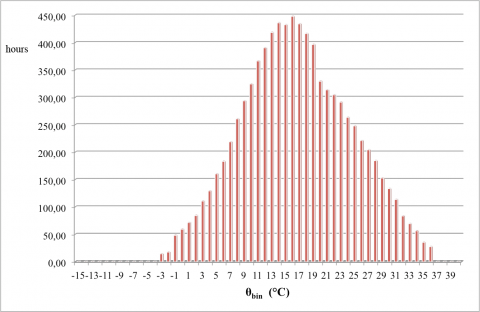Figure 2. Annual subdivisions monthly bin for Cosenza

4. Dimensioning with Ashrae Method

On the basis of the thermo-physical characteristics of the ground detected by on-site perforations (Table 1), we proceeded to the evaluation of its properties, bearing in mind also the UNI 13370: 2008 , for use in calculations.

Table 1. Thermo-physical characteristics of the soil found in situ

 Material Δz [m] u [kg/kg] λg [W/(m K)] ρg [kg/m3] ρg∙cg [kJ/(m3 K)] Peat 12 1.00 0.35 750 3,963 Clay 28 0.30 1.15 1,400 3,156 Silt 37 0.20 1.50 1,600 2,938 Wet sand 43 0.14 2.10 1,900 2,166

The average values obtained and used in the calculations are given in Table 2.

Table 2. Thermo-physical characteristics of the soil used for the calculations

 λg [W/(m K)] αg [m2/gg] ρg∙cg  [kJ/(m3 K)] 1.518 0.0466 2,814.771

The calculation of the undisturbed ground temperature makes reference to equation (11). Its implementation with MatLab software, allowed a high and sufficiently reliable resolution to be obtained. The undisturbed ground temperature was Tg = 16.58 °C. Once defined the identifying parameters of the ground, one can proceed in the calculation of the total length required in order to satisfy the thermal load. The thermal resistance of the Rgr filling is calculated by adopting the dual configuration U. The thermal resistance of the probe resulting from the calculation is equal to: Rb = 0.05 (m K)/W. The inlet and outlet temperatures (Twi and Two) can be chosen, based on common heat pumps available on the market in order to ensure their functioning in the design conditions. The operating temperatures are shown in Table 3.

Table 3. Flow and return temperatures of the Heat Pump

 Heating Cooling Twi  [°C] 5 35 Two [°C] 10 30

Considering the need to meet a peak load of about 180 kW during cooling, the choice fell to using 3 HPs model WRL200 AERMEC in parallel to be able to provide individually a cooling capacity of 64 kW. It was decided to use three heat pumps in parallel in order to optimize fuel consumption through the simultaneous functioning of the latter only in peak condition. During the winter season only two machines will be used, avoiding the reduction of the capacity control efficiency because of partial loading. In addition, the reversibility guarantees the possibility of working in both summer cooling and winter and used as the refrigerant R410a. Table 4 shows the main data of the data sheet of the heat pump.

Table 4. Technical data of Aermec WRL200° Heat Pump

 Thermal Power [kW] 69 Electrical power absorbed [kW] 17.46 COP 3.97 Cooling Power [kW] 64 Electrical power absorbed [kW] 14.52 EER 4.42

From the calculation of the total energy needs of the summer and the winter season, it is a project winter load Qh = 116 kW and a summer project load Qc = 177 kW.

The equivalent of a fully loaded annual operating hours for heating are τa,h = 424 h/year while the full load operating hours for cooling are τa,c = 853.49 h/year. The design loads were subsequently corrected by the use of the COP/EER of the machine:

$Q_{l c}=Q_{c} \cdot\left(1+\frac{1}{E E R}\right)=217,59 k W$(22)

$Q_{l h}=Q_{h} \cdot\left(1-\frac{1}{C O P}\right)=86,90 \mathrm{kW}$(23)

The calculation of the heat demand according to the Technical Specification UNI/TS 11300-1 takes into account the climate zone and the heating period imposed by the regulations. a 10-hour turn-on time per day was considered for 5 days per week within the actual use of the building. The partial monthly load factors in the months of the project amounted to:

PLFm,h = 0.238

PLFm,c = 0.247

Table 5 shows the project data relative to the heat exchanger in the ground.

Table 5. Characteristics of exchanger ground

 Probe Type Double U Number parallel systems 6 Thermal conductivity of the pipe λp [W/(m K)] 0.4500 Pipe outer diameter dpo [m] 0.0400 Thickness of the pipe S [m] 0.0037 Internal diameter of the pipe dpi [m] 0.0326 Wheelbase between the pipes d [m] 0.0600 Thermal conductivity filler λgr [W/(m K)] 0.7500 Drilling diameter db [m] 0.2000 Spacing between probes ds [m] 5.0000

The first of the probes of length values for the winter season and the summer were calculated by imposing a penalty temperature nothing. The values obtained are shown in Tables 6 and 7. Comparing the two results can be seen in a significant difference in length of the probes between the summer cooling and winter heating, owing to the strong load difference from having to meet.

The value obtained is however not satisfactory because it was obtained with a strong approximation. The penalty temperature, in fact, cannot be considered anything especially following the imposition of an interaxis between the probes of just 5 m. In addition to the number of probes in parallel, necessary to define the total number of them in order to hypothesize the arrangement, i.e., the factors N1, N2, N3, N4 present in eq. 14; the application of an iterative method, such as that of bisection, applied to air conditioning in summer will allow to find the point of correspondence between the final value and that imposed by applying a limited number of iterations. It has chosen to use an error of 0.0001 and a number of probes of 42.

The considered valid value for the satisfaction of the summer thermal needs of the building amounts to 4764 m divided into 42 probes from 113.43 m each of which yields an efficiency of land of 45.6 W/m (Table 8).

That provision cannot be considered the best unless after careful economic evaluation. Greater penetration depth leads to higher costs for single probe, lower total number of probes and improved efficiency (reduction of penalty temperature), which reduce the payback time.

The possible reduction of the number of probes would also be accompanied by an increase in the Reynolds number in that, for a defined section, would generate an increase of the flow rate, or speed.

Table 6. Dimensioning for winter heating

 Winter Heating Length probes for heating Lh [m] 1,391.18 Average annual power ground Qa [W] -22,224.59 Thermal load Qlh [W] 86,900.89 Capacity Factor PLFm,h [-] 0.24 Factor of short-circuit Fsc [-] 1.03 Thermal resistance probe-ground Rb [(m K)/W] 0.05 Resistance for annual pulse Rga [(m K)/W] 0.24 Resistance for monthly pulse Rgm [(m K)/W] 0.19 Resistance for day pulse Rgd [(m K)/W] 0.13 Undisturbed ground temperature Tg [°C] 16.58 Flow temperature Twi [°C] 5 Return temperature Two [°C] 10 Penalty temperature Tp [°C] 0

Table 7. Dimensioning for summer air conditioning

 Summer air conditioning Length probes for cooling Lc [m] 4,140.22 Average annual power ground Qa [W] -22,224.59 Heat load Qlc [W] -217,594.86 Capacity factor PLFm,c [-] 0.25 Factor of short-circuit Fsc [-] 1.03 Heat resistance probe-ground Rb [(m K)/W] 0.05 Resistance for annual pulse Rga [(m K)/W] 0.24 Resistance for monthly pulse Rgm [(m K)/W] 0.19 Resistance for day pulse Rgd [(m K)/W] 0.13 Undisturbed ground temperature Tg [°C] 16.58 Flow temperature Twi [°C] 35 Return temperature Two [°C] 30 Penalty temperature Tp [°C] 0

Table 8. Finding the value Tp and Lc by the bisection method

 Length field probes Lc [m] Resulting probe length l´ [m] Probe Number Penalty Temp. Tp [°C] hypothesiz. probe length l [m] Error 4,140.2168 98.5766 42 0.0000 0.0000 9.86E+01 4,874.9580 116.0704 42 -2.3994 98.5766 1.75E+01 4,747.9643 113.0468 42 -2.0378 116.0704 -3.02E+00 4,766.6794 113.4924 42 -2.0923 113.0468 4.46E-01 4,763.8492 113.4250 42 -2.0841 113.4924 -6.74E-02 4,764.2756 113.4351 42 -2.0853 113.4250 1.02E-02 4,764.2113 113.4336 42 -2.0851 113.4351 -1.53E-03 4,764.2210 113.4338 42 -2.0852 113.4336 2.31E-04 4,764.2195 113.4338 42 -2.0852 113.4338 -3.48E-05
5. Steady State Simulation of The Geothermal Field

The area available for the installation of geothermal probes is not extensive, and for this reason was initially carried out an analysis of stationary type, which of course, does not provide highly reliable results but are used for an initial rough assessment. Furthermore, in the case of geothermal probes, such an analysis must be performed for a very long time (the system can be considered next to quasi-steady), perform stationary analysis using algebraic equations for the calculation of the heat flow to assess the effects interference between the probes, it is always useful to be able to assess whether the available area is sufficient for the plant to heat pumps.

The MatLab software allows you to make two-dimensional field simulation using the finite element method through the application “PDE”. Figure 3 shows the geometry of the probes to simulate. Eight elements are visible corresponding to the 4 probes placed at the corners of a square and the respective analysis domains. The simulation space has been imposed as the sum of the surfaces represented by E1, E2, E5, E6. The modeling domain can be made on the basis of an elliptical or parabolic equation. The second type was chosen as it offers greater precision.

## figure_3.pngFigure 3. Geometry used for the evaluation of interference between the probes 5 m apart

The effect of interference between the probes is visible in Figure 4. The temperature in the middle point of the side generated between two successive wells reaches the value of about 28 °C, or about 12 °C more than the value associated with the undisturbed ground. The vectors representing the heat flow show the inefficiency generated. If this simulation had been made to the configuration of 6 x 7 probes, it would obtain a loss of thermal gradient of 38 % of the initial value of more than 50 % of the geothermal field. This simulation shows well the effects of the factor considered.

## figure_4.pngFigure 4. Temperature profile near 4 probes with a distance of 5 m apart

6. Geothermal Field Simulation with Trnsys

The analysis in dynamic mode allows all the parameters of the problem to be defined as a function of time. This analysis is very important in the simulation of the geothermal plant behavior because it provides more reliable and accurate results. The simulations in dynamic mode allows a more realistic condition of heat exchange with the ground to be obtained by assessing in detail the contributions from temperature increase or reduction due to the thermal inertia of the soil of the site itself and be subject to the variability of the weather conditions outside and thermal loads.

For the dynamic simulation of the geothermal field the software TRNSYS v.17 was used, and in particular the TYPE 557° block, specifically for field simulations of geothermal probes. The software model the soil according to the composition of the various layers placed and calculates the temperature by referring to the heat capacity, i.e. the inertia that characterizes it. The simulation spreading over the whole depth of penetration allows, if it were available, the insertion of the accurate data obtained through the execution of a GRT.

Among the output data provided by the software are of particular interest, the average temperature of the soil and the fluid outlet temperature, an important parameter for assessing the effects of the plant on the thermal state of the soil. The long-term effects could alter the temperature of the undisturbed ground implying alteration of the flora and a reduction of the energy produced. The simulations carried out with the software lead to identifying the best heat exchange efficiency. The maximum load condition is supposed each time the system is activated by performing the only limitation of power on public holidays.

The parameters relating to the characteristics of the terrain, the diameter of the probe, the diameter of the wells, flow, and distance characterizing the double U-shaped configuration are therefore considered to be always constant. The simulations were performed varying the wheelbase value, or the overall length of the probes, on a ten year basis to observe the influence of the long period of the field of the probes on the ground. Figure 5 shows the thermal load trend taken from the soil in the course of 10 years.

Different configurations were assumed by varying the depth of penetration into the soil and the subdivision in series and parallel. A total length of 4,800 m was calculated imposing a distance of 5 m. The configurations used for the simulations are shown in Table 9.

## figure_5.png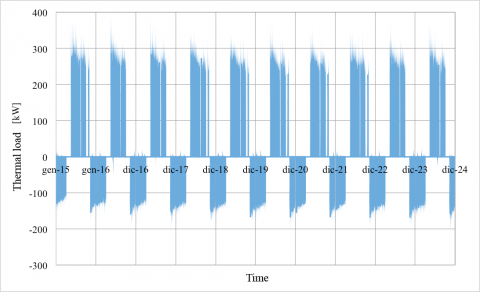Figure 5. Thermal load results taken from the ground during 10 year

Table 9. Configurations of the probes used for field simulation with a distance of 5 m

 Total Length [m] 4,800 Probes wheelbase [m] 5 Vt [m3] 103,908.17 N° probes Length [m] Parall. Series [kg/s] [kg/s] vf[m/s] 32 150 4 8 2.46 1.52 1.261 32 150 8 4 1.08 0.76 0.630 32 150 16 2 0.54 0.38 0.315 48 100 4 12 2.16 1.52 1.261 48 100 8 6 1.08 0.76 0.630 48 100 16 3 0.54 0.38 0.315 64 75 4 16 2.16 1.52 1.261 64 75 8 8 1.08 0.76 0.630 64 75 16 4 0.54 0.38 0.315

Figure 6 shows the trend comparison of the average temperature of the soil in the course of 10 years to 32 probes in the configuration of 2 series of 16 probes in parallel, of 4 series of 8 probes in parallel and of 8 sets of 4 probes in parallel. After the fifth year of use, the temperature settles at around 18.7 °C regardless of its current configuration. Figure 7, instead, shows the trend of the fluid outlet temperature from field probes, the probes for the different field configurations.

Figure 8 shows the change in the average temperature of the ground for 10 years for a field of 32, 48 and 64 probes, while Figure 9 shows the trend of temperature of the fluid in output from the geothermal field profile for two different field configurations of the probes.

## figure_6.png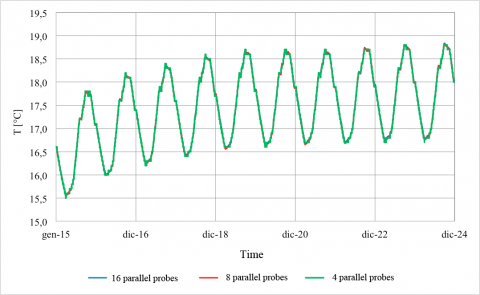Figure 6. Change in the average ground temperature for 10 years for 32 field probes with different configurations

## figure_7.png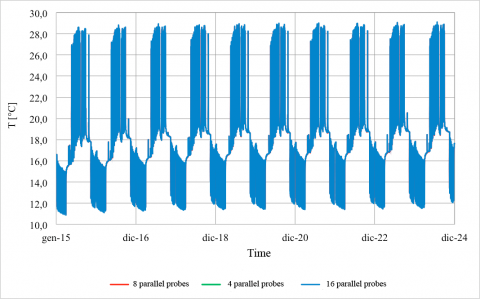Figure 7. Variation of the fluid outlet temperature for three different probes of the field configurations

## figure_8.pngFigure 8. Change in the average temperature of the ground for 10 years for a field of 32, 48 and 64 probes

## figure_9.png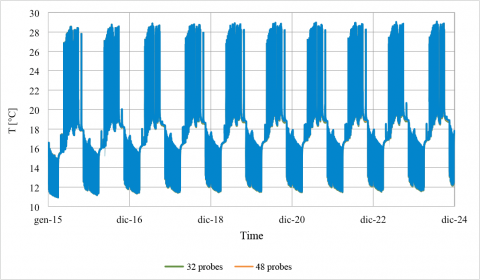Figure 9. Temperature of the fluid in output from the geothermal field profile for two different field configurations of the probes

As a result of the results obtained for the field probes with 5 m spacing, it was noticed that the different series / parallel configurations of probes, provide minimal effect on the average temperature of the soil after 10 years, it seemed appropriate to investigate the behavior of the field probes to vary the distance between the same. Imposing a distance between the probes of 7 m and 9 m were calculated the lengths necessary to meet the summer load of 4500 m and 4200 m respectively.

The simulations were carried out for several of the field configurations shown in Tables 10 and 11.

Table 10. Configurations of the probes used for the simulation field with distance 7 m

 Total Length [m] 4,400 Probes wheelbase [m] 7 Vt [m3] 190,931.27 N° probes Length [m] Parall. Serie [kg/s] [kg/s] vf[m/s] 30 150 5 6 1.73 1.22 1.009 30 150 10 3 0.87 0.61 0.504 30 150 15 2 0.58 0.41 0.336 45 100 5 9 1.73 1.22 1.009 45 100 9 5 0.96 0.68 0.560 45 100 15 3 0.58 0.41 0.336 60 75 6 10 1.44 1.02 0.841 60 75 10 6 0.87 0.61 0.504 60 75 15 4 0.58 0.41 0.336

Table11. Configurations of the probes used for field simulation with a distance 9 m

 Total Length [m] 4,200 Probes wheelbase [m] 9 Vt [m3] 294,579.7 N° probes Length [m] Parall. Series [kg/s] [kg/s] vf[m/s] 28 150 4 7 1.73 1.22 1.009 28 150 7 4 0.87 0.61 0.504 28 150 14 2 0.58 0.41 0.336 42 100 6 7 1.73 1.22 1.009 42 100 7 6 0.96 0.68 0.560 42 100 14 3 0.58 0.41 0.336 56 75 7 8 1.44 1.02 0.841 56 75 8 7 0.87 0.61 0.504 56 75 14 4 0.58 0.40 0.336

In Tables 9, 10 and 11, Vt is the volume of the solid matrix of the soil of which was taken into account in the simulations; and are the flow rates of the fluid for each of the U field probes for heating and cooling respectively and instead, Vf is the fluid velocity.

The simulations have led to similar results in terms of distance equal to the comparison between the probes, i.e., less effect on the ground in the case of smaller number of probes. A further comparison was performed by imposing a penetration depth of 150 m and 28 from field probes at 9 m distance, 32 to 5 m away probes and 30 probes to 7 m away. What did not appear evident in comparison with the same wheelbase, i.e. the temperature variation of soil in 10 years, it is clear by looking at the graph in Figure 10. We observe the influence of the wheelbase of the field probes that determines an asymptotic profile after 5 years of use, it characterized by a maximum value of 18.7 °C temperature with 5 m of distance up to a minimum temperature value of 17.7 °C with a distance of 9 m.

In Figure 10 the influence of the wheelbase of the field probes that determines an asymptotic profile after 5 years of use can be observed, it is characterized by a maximum value of 18.7 °C temperature with 5 m of distance up to a minimum temperature value of 17.7 °C with a distance of 9 m. What is of greater interest, however, is the maximum excursion of soil temperature after 5 years. Recall that the use of the land is annual and that the maximum excursion reaches 2 °C for a center distance of 5 m and 0.6 °C for center distance of 9 m (Figure 11).

Similar studies were conducted by using TRNSYS for horizontal probes geothermal fields obtaining similar results .

7. Analysis of Results

From the values obtained as a result of the simulations, it is easy to deduce the most relevant parameters available in the field of geothermal probes. An analysis of the results obtained, it appears that:

The number of parallel circuits: does not affect the long-term effects generated on average constant temperature of the ground, i.e., on the temperature difference generated on the fluid which remains unchanged.

## figure_10.png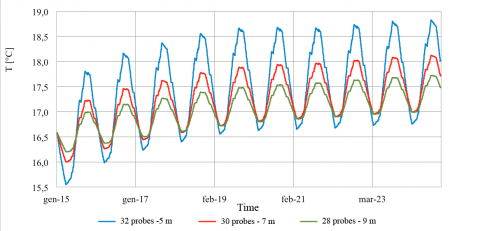Figure 10. Change in the average temperature of the ground at variation the wheelbase of the field probes

## figure_11.png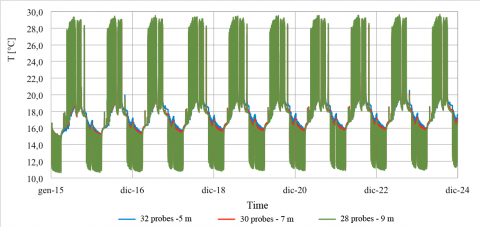Figure 11. Temperature of the fluid in the output profile from the geothermal field for different configurations of the field probes to variable distance

The number of probes and the length of the probes: for the soil stratigraphy considered and for the effects of the locations of the project temperatures, it appears that a smaller number of probes characterized by a greater length reduces the effect of thermal drift of the terrain ensuring an operation for a greater number of years;

The center distance between the probes: the increase of the distance between the wells generates the effect of greater relevance. The increase of the distance between the probes reduces the penalty for interference effects and increase of the temperature of the ground allowing a greater heat transfer to the fluid in output from the sensor field.

The calculated changes are not an important parameter for the choice of one or the other configuration which, however, will have to be defined on the basis of a detailed economic analysis.

8. Conclusions

The hypothesis of using an HPs air/water to satisfy the thermal load of the project does not allow to meet the dictates of the Legislative Decree no. 28/2011 on the share of renewable energy. Moreover, it should provide for the presence of a boiler and a cooling machine of integration to meet the requirements of heating and cooling of the building. The use of geothermal heat pumps is a viable alternative for a significant share of renewable energy, as the calculations show that the temperature of the land after 10 years of use does not exceed 18.7 °C for all the simulated configurations.

By sizing the probes field run with the ASHRAE method a total length of variable probes between 4200 m and 4800 m was adopted according to wheelbase theorized. Taking into consideration the cost items and the long-term performance, since it minimizes the thermal drift of the ground, it can be said that the optimal solution is to use probes 28 to 150 m depth each. The validity of this solution was also confirmed by the simulations with TRNSYS software from which the fluid return to the HPs temperature is always maintained below 30 °C required with a further improvement of the performance of the machine. This choice has as limitation the fact that the total area required passes from 800 m2 with the configuration of the probes 32 to 5 m to 1,372 m2 wheelbase in the configuration of the probes 28 to 9 m by 150 m of depth each.

Also, it is very important to investigate the economic impact of ground-source heat pump for heating and cooling in comparison with traditional systems .

Nomenclature
 d wheelbase between the pipes, m db drilling diameter, m ds spacing between probes, m dpo pipe outer diameter, m dpi internal diameter of the pipe, m cg ground specific heat, J.kg-1. K-1 COPh average seasonal values of the coefficient of performance of the heat pump in heating mode COPc average seasonal values of the coefficient of performance of the heat pump in cooling mode Fo Fourier number Fsc Factor of short-circuit Gf G-factor Lh total length of the probe field for heating, m Lc total length of the probe field for cooling, m N1 .. N4 number of probes Ntot total number of probes PLFm,c/h capacity factor Qa average annual power exchanged with the ground, W Qacc heat accumulated after 10 years, W Qlc thermal design load for cooling, W Qlh thermal design load for heating, W Rb thermal resistance probe-ground,  m.K.W-1 Rga equivalent probe-ground thermal resistance per unit length for annual pulse, m.K.W-1 Rgm equivalent probe-ground thermal resistance per unit length for monthly pulse, m.K.W-1 Rgd equivalent probe-ground thermal resistance per unit length for daily pulse, m.K.W-1 Rgr thermal resistance probes-soil, m.K.W-1 Rpp overall thermal resistance of the pipes,    m.K.W-1 S thickness of the pipe, m T period of the sinusoidal pulse, s Tg undisturbed ground temperature, °C Tgeo geothermal temperature gradient, °C Twi inlet temperature of the fluid that feeds the geothermal probes in the design conditions, °C Two outlet temperature of the fluid that feeds the geothermal probes in the design conditions, °C Tp penalization temperature, °C T0 average annual temperature of the locality, °C ΔT0 variation of the amplitude of the sine wave of the surface temperature,  °C t0 number of the day, dd Vf fluid velocity, m.s-1 Vt volume of the solid matrix of the soil, m3 Wc electric power absorbed by the compressor of the heat pump in cooling mode, W Wh electric power absorbed by the compressor of the heat pump in heating mode, W

Greek symbols

 αg thermal diffusivity of the ground, m2.s-1 δ depth of penetration of heat wave, m λp thermal conductivity of the pipe, W.m-1. K-1 λg thermal conductivity of the ground, W.m-1. K-1 λgr thermal conductivity of the hole fill material,   W.m-1. K-1 ρg density of the ground, kg. m-3 τ period characterizing the impulse, s τa,h operating hours for heating, h τa,c operating hours for cooling, h ω heat wave pulse, s-1

Subscripts

 a average seasonal value b probe c cooling g ground ga annual gd daily gm monthly h heating p penalty wi inlet flow wo outlet flow
References

 ASHRAE Handbook, “HVAC Applications,” SI Edition, Atlanta (USA), 2007.

 TRNSYS 17. Thermal Energy System Specialists, LLC 22 North Carroll Street, Suite 370 Madison, WI 53703 USA.

 UNI 11466:2012, “Sistemi geotermici a pompa di calore - Requisiti per il dimensionamento e la progettazione,” 2012.

 L. R. Ingersoll, O. J. Zobel and A. C. Ingersoll, Heat Conduction with Engineering and Geological Application, 2nd ed., McGraw Hill, New York, 1954.

 S. P. Kavanaugh and K. Rafferty, “Ground source heat pumps - Design of geothermal systems for commercial and institutional building,” ASHRAE Applications Handbook, Atlanta, 1997.

 H. S. Carslaw and J. C. Jaeger, Conduction of Heat in Solids, Oxford at the Clarendon Press, 1959.

 A. Zarrella, “L’uso del terreno come sorgente termica,” Dipartimento di Fisica Tecnica – Ed. Università degli Studi di Padova, 2009.

 G. Hellström, “Ground heat storage. Thermal analysis of duct storage systems. I Theory,” University of Lund, Department of Mathematical Physics, Lund, Sweden, 1991.

 UNI TS 11300-4:2012, “Prestazioni energetiche degli edifici - Parte 4: Utilizzo di energie rinnovabili e di altri metodi di generazione per la climatizzazione invernale e per la produzione di acqua calda sanitaria,” 2012.

 UNI EN ISO 13370:2008, “Prestazione termica degli edifici - Trasferimento di calore attraverso il terreno - Metodi di calcolo,” 2008.

 M. Bottarelli, “Numerical Analysis of Heat Transfer induced by an Horizontal Ground Heat Exchanger in an Heterogeneous Soil,” International Journal of Heat and Technology, vol. 28, n° 2, pp. 137-142, 2010.

 M. Bottarelli and L. Gabrielli, “Payback period for a ground source heat pump system,” International Journal of Heat and Technology, vol. 29, n° 2, pp. 145-150, 2011.